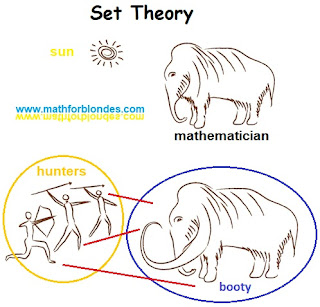## 8/09/2019

### Set theorySet Theory

Set theory is the stone age of mathematics. Only shamans know how to properly form the set. Let's look at set theory from the side and reveal some of the secrets of shamans. To understand the essence of set theory, it is necessary to consider another mathematical concept - a function. The function sets the correspondence between the elements of two sets. Each element from the herd of hunters is associated with one or more elements from the herd of prey, each element from the herd of prey is assigned one or more elements from the herd of hunters. Only shamans know what kind of herd belongs to and how to divide prey properly.

What is set theory? This is a herd theory. What will happen if we combine a herd (set) mathematicians and a herd (set) sheep: sheeps with a mathematical education or mathematicians with sheep’s brains? I don’t know what set theory says about the results of such a union, but in reality mathematicians will have a great barbecue picnic. I have nothing against set theory, as one of the mathematical tools. But to stand up for “scientific” theories based on such a primitive and vague concept is already too much.

Any theory should be tested by practice, even mathematical. I will show you an example of the practical application of set theory by the example of such concepts as “set” and “multiset”.

1.Yes, thanks for this one.

2.Set theory in general deals with sets of elements and their characteristics as well as operations and relations of those sets. It's a fundamental way to group things of similar properties. Sets are important because they encode a totality of information of a certain kind, more formally. Set theory is also scoring topics in mathematics. For learning maths, you just have to find the best mathematics tutor who can clear your concept & make your basics strong.

1.Aliens teach me math. That is the problem.

3.Sandy Hook Parent